# How to Count Characters in a Excel cell or range

Feb 23, 2020 • edited Feb 24, 2020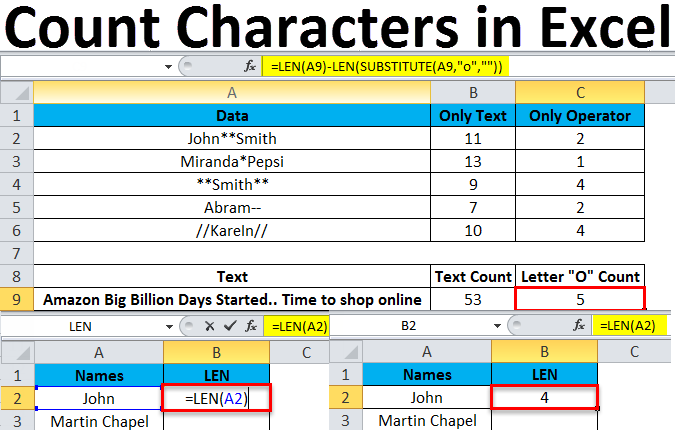# Count Characters

To count the number of a specific character in a single cell of a list may be easy when there only several cells. However, if there are dozens of cells in a list, how can you quickly count a specific character in each cell of the list in Excel?

Be a genius with Excel in this tutorial of How to count characters in Microsoft Excel. Easy formula, easy drag formula repeat function.

Learn how to count the number of characters in a cell or range of cells and how to count how many times a specific character occurs in a cell or range of cells.

1. The LEN function in Excel counts the number of characters in a cell.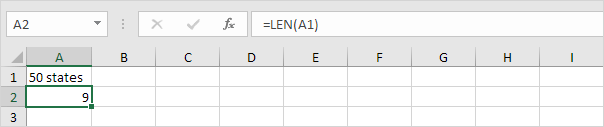Explanation: the LEN function counts 2 numbers, 1 space and 6 letters.

2. You can combine the SUM function and the LEN function to count the number of characters in a range of cells.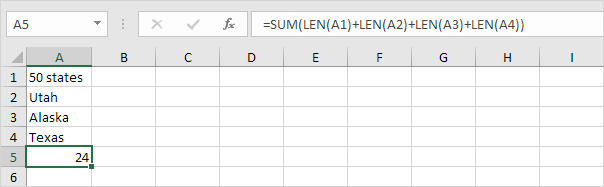Note: as you can imagine, this formula can get quite long.

3. The array formula below counts the number of characters in a range of cells.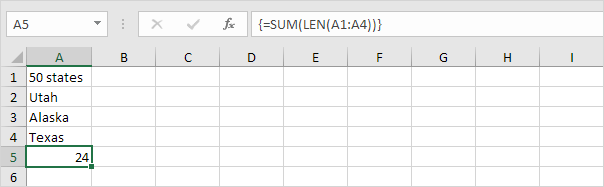Note: finish an array formula by pressing CTRL + SHIFT + ENTER. Excel adds the curly braces {}. The array constant {9;4;6;5} is used as an argument for the SUM function, giving a result of 24. Maybe this is one step too far for you at this stage, but it shows you one of the many other powerful features Excel has to offer.

4. Use the LEN function and the SUBSTITUTE function to count how many times a specific character (in this example, the character a) occurs in a cell.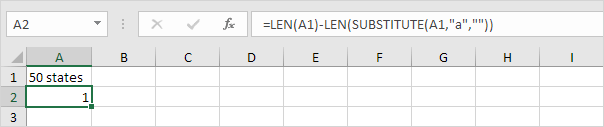Explanation: the SUBSTITUTE function replaces the character a (second argument) with an empty string (third argument). LEN(SUBSTITUTE(A1,“a”,"")) equals 8 (the length of the string without the character a). If we subtract this number from 9 (total number of characters in cell A1), we get the number of occurrences of the character a in cell A1.

5. The array formula below counts how many times a specific character (in this example, the character a) occurs in a range of cells.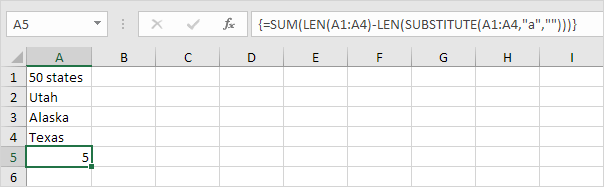Note: finish an array formula by pressing CTRL + SHIFT + ENTER. Excel adds the curly braces {}. The array constant {1;1;2;1} is used as an argument for the SUM function, giving a result of 5. The SUBSTITUTE function is case-sensitive. The A in Alaska is not counted.

6. The array formula below counts both lower and upper case occurrences of a specific character (in this example, the character a).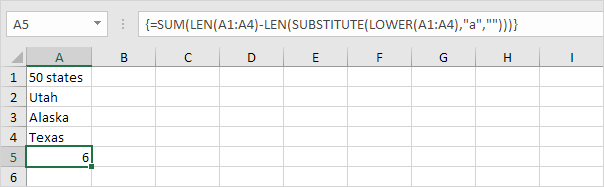Explanation: The LOWER function converts all letters to lowercase first.

#Tutorial#How To#Functions

How to Count Cells with Text in Excel

How to Count Blank and Non Blank Cells in Excel